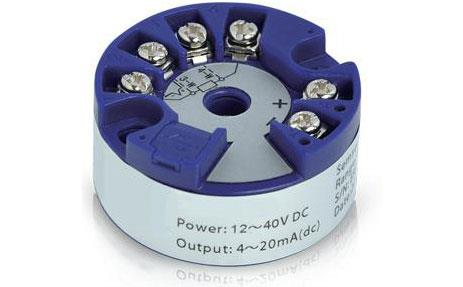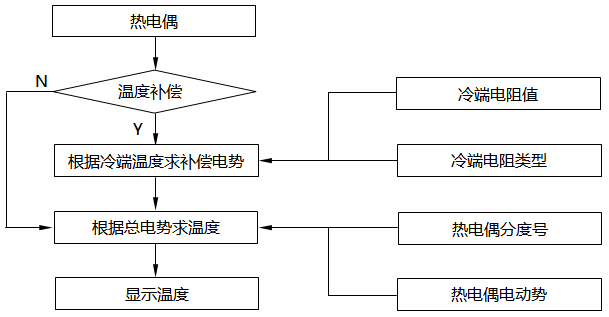﻿ 智能温度变送器信号处理软件算法与软件设计

# 智能温度变送器信号处理软件算法与软件设计

2019/9/19 11:38:24 人评论 次浏览 分类：变送器  文章地址：http://yunrun.com.cn/tech/1175.html1、软件流程方案

2、热电阻传感器算法

Strcmp(category,″pt10″)==0；//category[]是电阻类型数组。

3、热电偶冷端补偿算法

Horner算法是计算多项式函数值的标准化算法，程序设计中由冷端温度值推算出冷端补偿电势的过程中，使用Horner规则节省多项式的计算量。

4、热电偶传感器综合算法If(compensate==1)
{
Ctemp=resistanceTemp(ccategory,rcvalue)；
Cpotential=coldCompensate(ctemp,ccatego-ry)；
Value+=cpotential；
}
Temp=(float)couple2Temp(category,value)；
Returntemp;

表1  空间统计
 输入信号类型 系数个数 占用空间/Byte R 11+10+6+5=32 256 S 10+10+6+5=31 248 B 9+9=18 144 J 9+8+6=23 184 T 8+7=15 120 E 9+10=19 152 K 9+10+7=26 208 N 10+8+6=24 192 合计 188 1504

5、结束语

 与在线工程师QQ交流 到论坛与网友交流 向昌晖仪表网投稿 免费获得2000元电子书

客户姓名：